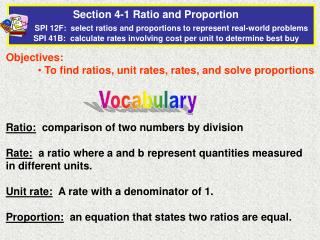Download PresentationObjectives: To find ratios, unit rates, rates, and solve proportions

# Objectives: To find ratios, unit rates, rates, and solve proportions - PowerPoint PPT PresentationDownload Presentation## Objectives: To find ratios, unit rates, rates, and solve proportions

- - - - - - - - - - - - - - - - - - - - - - - - - - - E N D - - - - - - - - - - - - - - - - - - - - - - - - - - -
##### Presentation Transcript

1. Section 4-1 Ratio and ProportionSPI 12F: select ratios and proportions to represent real-world problems SPI 41B: calculate rates involving cost per unit to determine best buy • Objectives: • To find ratios, unit rates, rates, and solve proportions Vocabulary Ratio: comparison of two numbers by division Rate: a ratio where a and b represent quantities measured in different units. Unit rate: A rate with a denominator of 1. Proportion: an equation that states two ratios are equal.

2. Ratio (comparison of 2 numbers by division) The ratio of two quantities a and b, can be written as: a to b a:b Example What is the ratio of girls to boys if there are 13 girls and 22 boys? 13 to 22 13:22

3. Real-world and Ratios In the plains of Africa, zoologist tagged zebras to discover that there exists 178 female zebras to 148 male zebras. What is the ratio of male to total number of zebra? There are 148 male zebras. There is a total of 326 zebras. The ratio is 148 to 326 148:326 Always reduce to lowest terms 74 to 163 74:163

4. Rate (Ratio where a and b represent quantities measured in different units) Unit Rate Rate with a denominator of 1 Since your income is limited, you want to shop for the best bargains in a grocery store. You are purchasing apple juice. The price of apple juice is \$0.72 for 16 oz or \$0.90 for 18 oz. Which is the better buy? Shopping \$0.72 for 16 oz \$0.90 for 18 oz This one is the better buy.

5. Real-world and the Unit Rate How much does a person earn if they work 50 hours and receive \$475? You have paid \$44 to skate at a skateboard park for 8 hours. What is the cost per hour (unit rate)?

6. Proportions (an equation that states two ratios are equal) Cross Products of Proportions (Use ONLY when one ratio or fraction equals another) Use Multiplication Property of Equality to Solve Proportions

7. Use Cross-Products to Solve the Proportions Do Now 1. Solve the proportion: 2. Solve the proportion: Distributive Property Simplify SPE Simplify SPE Simplify

8. Setting up to Write a Proportion If people could jump as high as a flea, how high could a person, that is 5 feet tall jump if a flea that is .125 inches tall can jump to a height of 13 inches? Jump height Tallness 13 .125 x 5 = Answer the question A person could jump to a height of 520 feet.

9. 3630 92.5 295 t = 3630t = 92.5(295) Write cross products. 92.5(295) 3630 t = Divide each side by 3630. t 7.5 Simplify. Round to the nearest tenth. Write and Solve a Proportion Do Now In 2000, Lance Armstrong completed the 3630-km Tour de France course in 92.5 hours. Traveling at his average speed, how long would it take Lance Armstrong to ride 295 km? Traveling at his average speed, it would take Lance approximately 7.5 hours to cycle 295 km.